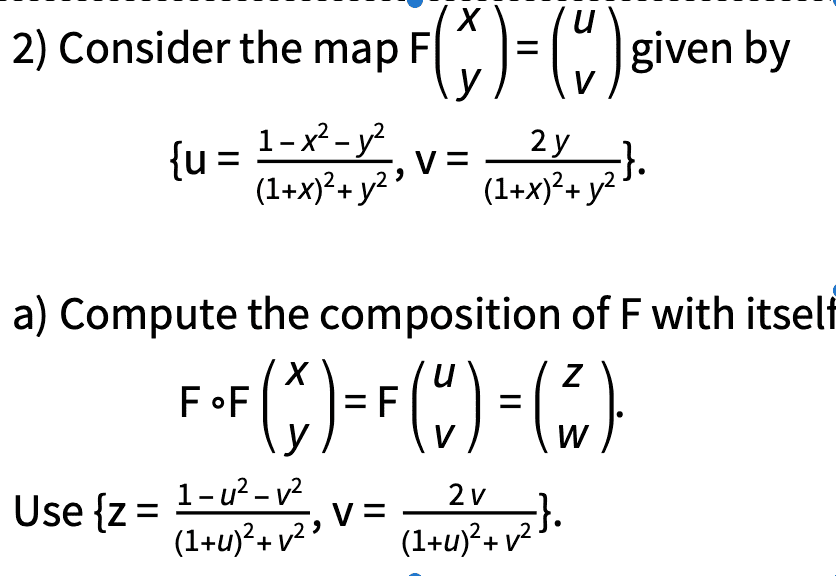# Find the composition of a multivariate function with itself

docnet
Homework Statement:
psb
Relevant Equations:
psbSolution attempt:

$$F(F(x,y))=(z,w)$$

is the map given by

$$x=z$$
$$y=w$$

•anuttarasammyak

mitochan
I observe for x=-1,y=0, u=0/0,v=0/0 not defined so this point should be excluded.

Mentor
2022 Award
Homework Statement:: psb
Relevant Equations:: psb

View attachment 278552

Solution attempt:

$$F(F(x,y))=(z,w)$$

is the map given by

$$x=z$$
$$y=w$$
No. The map is given if you express ##z=z(u,v)=z(u(x,y),v(x,y))=z(x,y)##. You have to substitute the ##u,v## with their definitions in terms of ##x,y##.

mitochan
You have to substitute the with their definitions in terms of .
I assume after the substitution he got this result
##F^2=E##
##F=F^{-1}##

Mentor
2022 Award
I assume after the substitution he got this result
##F^2=E##
##F=F^{-1}##
Maybe, but I guess there is no way but calculation to be sure. It looks a bit like a local Lie group.

mitochan
I should appreciate it if you would tell me what kind of transformation this ##F = F^{-1}## is. Named? Usage?

Mentor
2022 Award
It is called reflection, or involution.

mitochan
Thanks. And for this special idempotent transformation, is it used in Physics ?

Mentor
2022 Award
Thanks. And for this special idempotent transformation, is it used in Physics ?
I corrected it. Idempotent functions are ##F^2=F##. We have theoretically ##F^3=F## and any element with ##F^n=F## qualifies to be idempotent, but Wiki said idempotent functions are those with ##n=2##.

So let's call it reflection or involution. These terms already suggest their usage. Any reflection satisfies ##F^2=1:## reflected twice is the original. This is a geometric property but as such also used in physics whenever geometry plays a role (crystallography, particle physics, mechanics). Other examples for involutions are matrix transposition, complex conjugation or the Legendre transformation.

•docnet
docnet
As Fresh_42 was explaining, ##F## is a "Möbius transformation" at its core, an involution about a circle in an offset coordinate system. That's how I got my answer by thinking ##F=F^{-1}##. Although my answer is probably wrong, because ##F## is not defined everywhere on the ##uv## plane to start with. I wonder if the composite map would be different if I computed everything out?

This problem was assigned last semester in differential geometry by the person who invented the Ricci flow and was partially credited for solving the Poincaré conjecture. This was probably too difficult to solve for most undergraduate students in differential geometry.

•mitochan
Homework Helper
Gold Member
2022 Award
We can construct this transformation as follows. First, we have something simpler: $$u = \frac{2x}{x^2 + y^2}, \ \ v = \frac{2y}{x^2 + y^2}$$ Leads to the same inverse transformation: $$x = \frac{2u}{u^2 + v^2}, \ \ y =\frac{2v}{u^2 + v^2}$$ Now, if we let ##x' = x +1## and ##u' = u +1##, and we let:
$$u' = \frac{2x'}{x'^2 + y^2}, \ \ v = \frac{2y}{x'^2 + y^2}$$ With the same inverse transformation: $$x' = \frac{2u'}{u'^2 + v^2}, \ \ y =\frac{v}{u'^2 + v^2}$$ Then express that in terms of ##x, u## we have:
$$u +1 = \frac{2(x+1)}{(x+1)^2 + y^2}, \ \ v = \frac{2y}{(x+1)^2 + y^2}$$ With, again, the same inverse transformation. Finally, we re-express ##u##:
$$u = \frac{1 - x^2 - y^2}{(x+1)^2 + y^2}$$ And we see that it's the same transformation as above.

•mitochan
mitochan
Thanks @PeroK
Although my answer is probably wrong, because is not defined everywhere on the plane to start with. I wonder if the composite map would be different if I computed everything out?
The transformation for (x,y)=(-1,0) ## u(-1,0)=-1, v(-1,0)=0##, otherwise as above mentioned would be defined for all xy, uv plane.